Spontaneously Broken Symmetry in the Hubbard Model

Sho Yaida April 27, 2007

(Submitted as coursework for AP273, Stanford University, Spring 2007)

Spontaneous breakdown of symmetry is ubiquitous in physics. Crystallization breaks the translational symmetry of a fluid phase, while ferromagnetization breaks the rotational symmetry of a paramagnetic phase. Breakdown of gauge symmetry is important both in the BCS theory of superconductivity and in the Standard Model of particle physics.

Here, we shall examine a simple model showing this phenomenon.

Hubbard Model

The Hamiltonian for the one-band Hubbard model is given by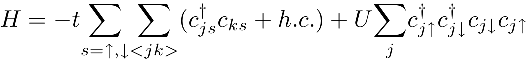where t and U are positive, and c+js (cjs) creates (annihilates) an electron of spin s at a lattice site j. < jk > denotes a sum over nearest-neighbor pairs of sites j and k. We shall assume a 2-dimensional square-lattice structure, along with a periodic boundary condition. Finally, we fix a number of electrons by the condition Ne=Nsite.

This model has a propensity for antiferromagnetization. The last term in the Hamiltonian, with our assumption Ne=Nsite, favors configurations with one electron per site. A little examination (see, e.g., ) shows that the other terms in turn try to anti-align neighboring electrons' spins. Thus, we expect antiferromagnetization for a macroscopic sample at low temperature.

Hartree-Fock Ansatz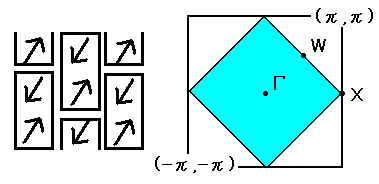Fig.1: Effectively bigger unit cells and effectively smaller Brillouin zone.

So, anticipating antiferromagnetization, we make the following ansatz: We assume that the "ground state" is given by filling up the vacuum with Bloch-orbitals constructed with respect to effectively bigger cells depicted in Fig.1, with the equal number of up and down spins. Mathematically, we use the basis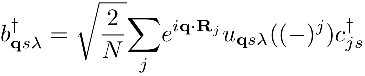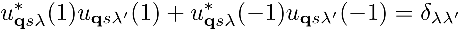to fill up the vacuum. q ranges over the effectively smaller Brillouin zone (Fig.1).

We determine the shapes of Bloch-orbitals by minimizing the resulting energy expectation value of the "ground state."

Resulting Order and Band Structure

A straightforward variational argument yields: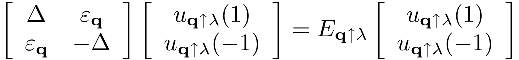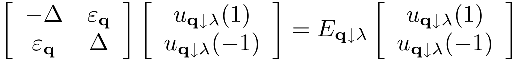where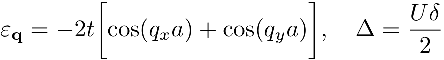Here, δ has the following relations with the "ground state" expectation values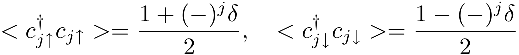and satisfies the following equation: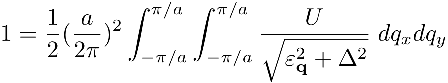Note that the "ground state" indeed has average directions of spins alternating from site to site, showing antiferromagnetization.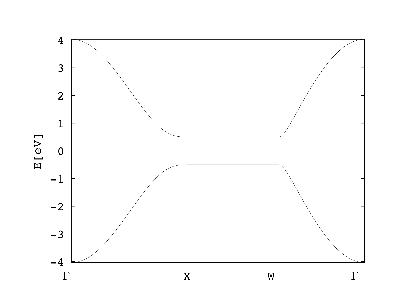Fig.2: Resulting band structure(for t=2Δ=1[eV]).

The band structure is dictated by: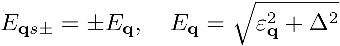and is depicted in Fig.2. (Click here for the fortran code and here for gnuplot.) The "ground state" is the one with the lower band filled. The exciton spectrum has an energy gap Egap=2Δ>0.

Concluding Remarks

Hidden in our ansatz is a huge amount of physics. Breakdown of symmetries can result from the interplay of thermodynamic limit making energy spectrum near the ground state degenerate and infinitesimal external field preferring one of the degenerate states. Or it can arise from thermodynamic limit making huge part of the Hilbert space unreachable from the initial state with which we start the system to equilibrate. However, it is hard to prove its occurrence except few special cases.

In our approach, we have implicitly put in the seed of antiferromagnetization by hand, and in no way have we derived it. This is also the case in mean-field approach (see, e.g., ). It is rather an assumption, from which further properties of the system are derived and compared with experiments. It is an excellent assumption, nevertheless.

© 2007 S. Yaida. The author grants permission to copy, distribute and display this work in unaltered form, with attribution to the author, for noncommercial purposes only. All other rights, including commercial rights, are reserved to the author.

Reference

 E. Fradkin, Field Theories of Condensed Matter Systems (Addison-Wesley, 1991).